Students can download 5th Maths Term 3 Chapter 6 Fractions 6.2 Questions and Answers, Notes, Samacheer Kalvi 5th Maths Guide Pdf helps you to revise the complete Tamilnadu State Board New Syllabus, helps students complete homework assignments and to score high marks in board exams.

## Tamilnadu Samacheer Kalvi 5th Maths Solutions Term 3 Chapter 6 Fractions 6.2

Question 1.
Write the suitable number in the box.
(i)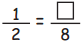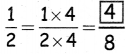(ii)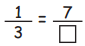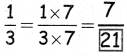(iii)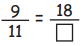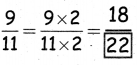(iv)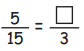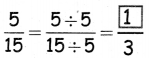(v)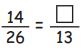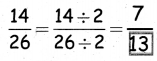(vi)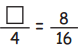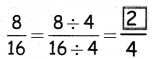(vii)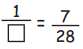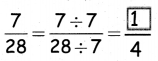(viii)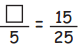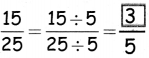Question 2.
Find an equivalent fraction with denominator 18 for each of the following fractions $$\frac{1}{2}, \frac{2}{3}, \frac{4}{6}, \frac{2}{9}, \frac{7}{9}, \frac{5}{3}$$
$$\frac{1}{2}=\frac{1 \times 9}{2 \times 9}=\frac{9}{18}$$
$$\frac{2}{3}=\frac{2 \times 6}{3 \times 6}=\frac{12}{18}$$
$$\frac{4}{6}=\frac{3 \times 4}{3 \times 6}=\frac{12}{18}$$
$$\frac{2}{9}=\frac{2 \times 2}{9 \times 2}=\frac{4}{18}$$
$$\frac{7}{9}=\frac{7 \times 2}{9 \times 2}=\frac{14}{18}$$
$$\frac{5}{3}=\frac{5 \times 6}{3 \times 6}=\frac{30}{18}$$Question 3.
Find an equivalent fraction with denominator 5 for each of the following fractions $$\frac{6}{15}, \frac{10}{25}, \frac{12}{30}, \frac{6}{10}, \frac{21}{35}$$
Answeer:
$$\frac{6}{15}=\frac{6 \div 3}{15 \div 3}=\frac{2}{5}$$
$$\frac{10}{25}=\frac{10 \div 5}{25 \div 5}=\frac{2}{5}$$
$$\frac{12}{30}=\frac{12 \div 6}{30 \div 6}=\frac{2}{5}$$
$$\frac{6}{10}=\frac{6 \div 2}{10 \div 2}=\frac{3}{5}$$
$$\frac{21}{35}=\frac{21 \div 7}{35 \div 7}=\frac{3}{5}$$